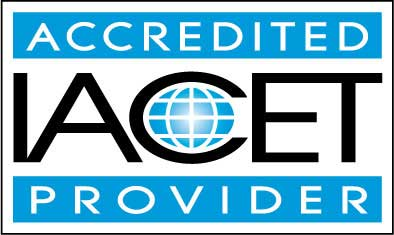# Course Syllabus## Course Description

Although we may not always recognize it, we use basic geometry skills regularly in everyday life. For instance, we consider whether a picture on the wall is parallel with the floor, or we calculate the area of a room when installing new carpeting.

Geometry is also an integral part of many other areas of study, including physics and other natural sciences. This course provides a more rigorous mathematical foundation for both practical and theoretical geometric thinking.

The course begins by considering basic figures such as points, lines, and planes, and then expands into the concepts of parallelism and perpendicularity as well as more complicated geometric figures such as polygons (triangles, quadrilaterals, and so on) and circles. The course considers mainly two-dimensional figures, but two lessons on three-dimensional geometry provide more complete coverage of the subject.

Each successive lesson in the course builds on previous lessons, culminating in lessons that teach the student how to calculate lengths, areas, and volumes of more complicated figures. In addition to its in-depth discussion of the mathematical aspects of geometry, the course also includes lessons on how to draw various figures using a compass and straightedge as well as a lesson on strategy for solving real-world geometry problems.

The course concludes by applying all that the student has learned to a brief introduction to trigonometry, thereby providing a jumping-off point for a more rigorous study of geometry as well as other areas of mathematics. By the end of the course, the student should feel comfortable tackling a range of basic to moderately difficult geometry problems.
• Completely Online
• Self-Paced
• 6 Months to Complete
• Start Anytime
• PC & Mac Compatible
• Android & iOS Friendly
• Accredited CEUs## Learning Outcomes

By successfully completing this course, students will be able to:
• Define basic geometry terms.
• Define geometric reasoning and measurement.
• Describe angles and parallelism.
• Describe properties of triangles.
• Describe congruent triangles.
• Describe similar triangles.
• Solve problems involving Polygons.
• Solve problems involving circles.
• Describe classical construction.
• Solve practical geometry problems.
• Describe three-dimensional geometry.
• Demonstrate mastery of lesson content at levels of 70% or higher.

## Assessment Guide

Assessment Points
Lesson 1 Assignment 1 points
Lesson 1 Geometry Terms and Motivation 10 points
Lesson 1 Exercises 5 points
Lesson 2 Geometric Reasoning and Measurement 10 points
Lesson 3 Angles and Parallelism 9 points
Lesson 4 Triangles I: Properties of Triangles 10 points
Lesson 4 Exercises 3 points
Lesson 5 Triangles II: Congruent Triangles 10 points
Lesson 5 Exercises 3 points
Lesson 6 Triangles III: Similar Triangles 7 points